# 这些一行 JS 实现功能的代码，让你看起来像一个前端专家

JavaScript 可以做很多神奇的事情！

## 1. 获取一个随机布尔值 (true/false)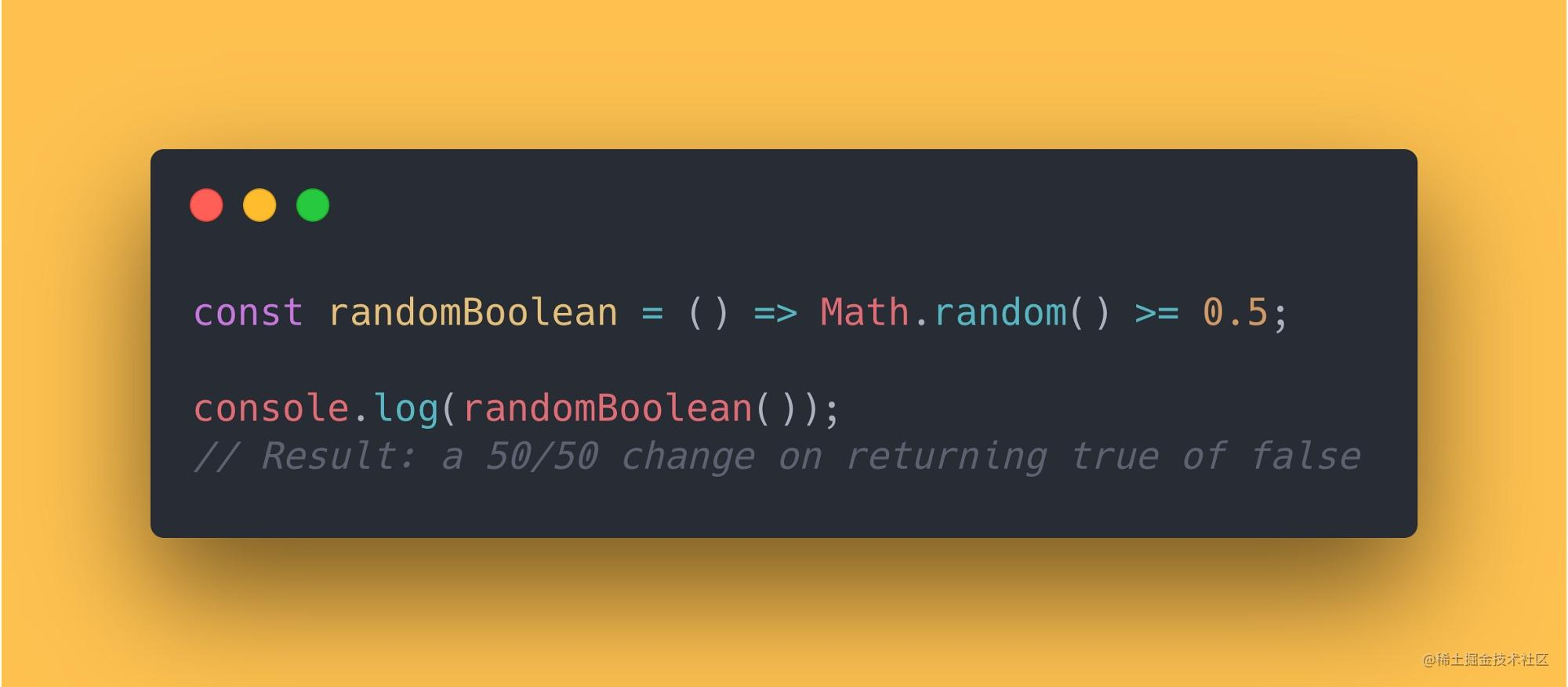``````const randomBoolean = () => Math.random() >= 0.5;
console.log(randomBoolean());
// Result: a 50/50 change on returning true of false

## 2. 检查日期是否为工作日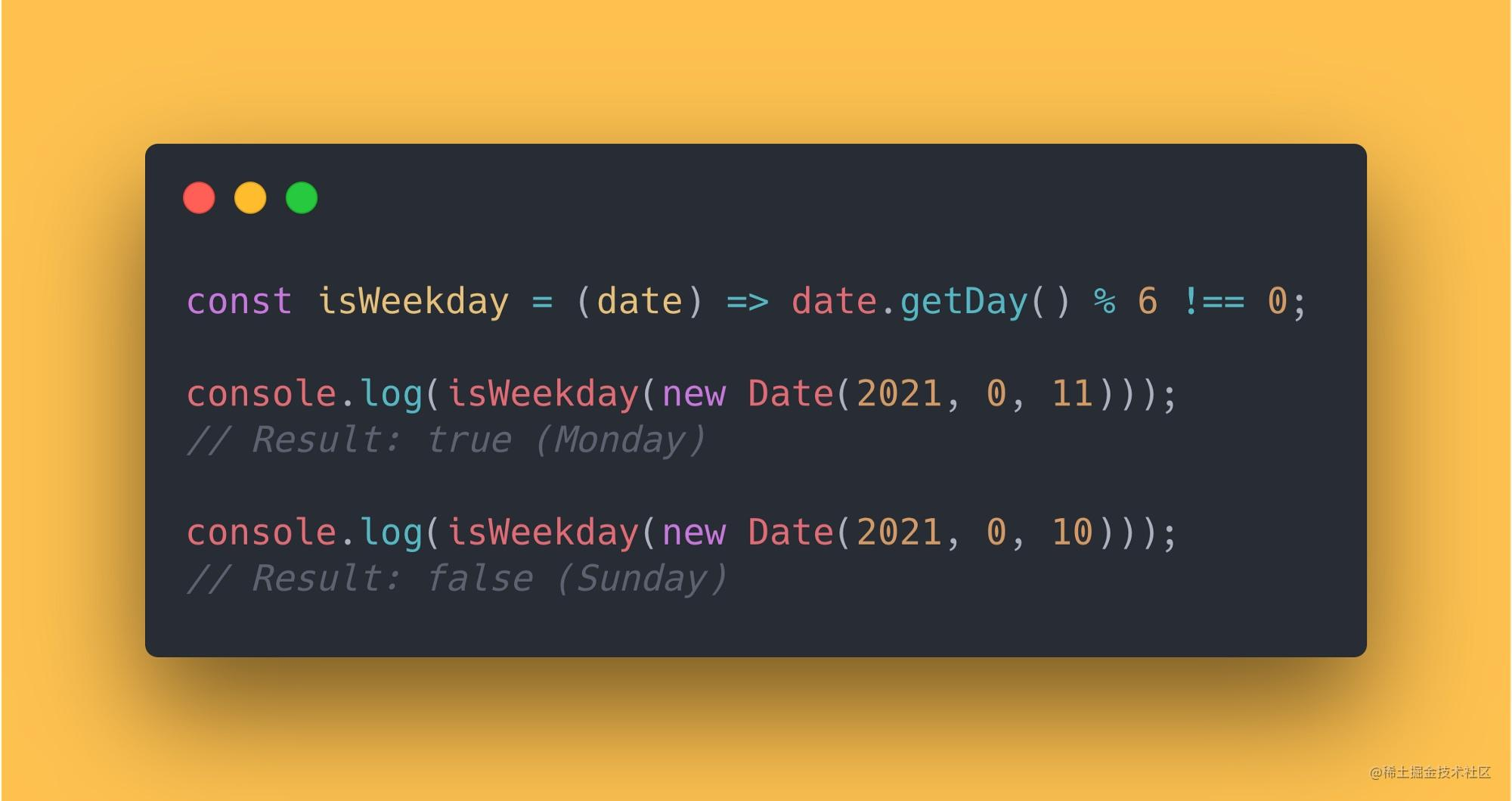``````const isWeekday = (date) => date.getDay() % 6 !== 0;
console.log(isWeekday(new Date(2021, 0, 11)));
// Result: true (Monday)
console.log(isWeekday(new Date(2021, 0, 10)));
// Result: false (Sunday)

## 3. 反转字符串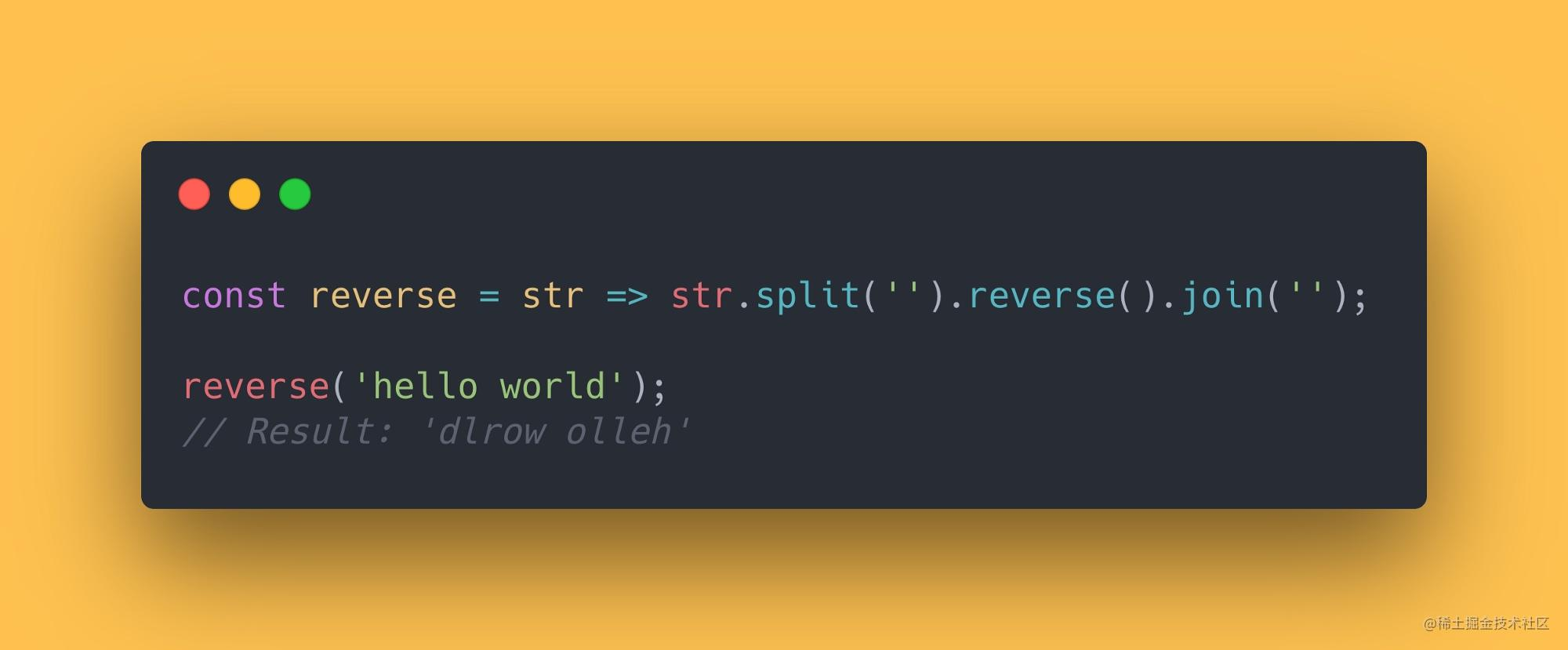``````const reverse = str => str.split('').reverse().join('');
reverse('hello world');
// Result: 'dlrow olleh'

## 4. 检查当前 Tab 页是否在前台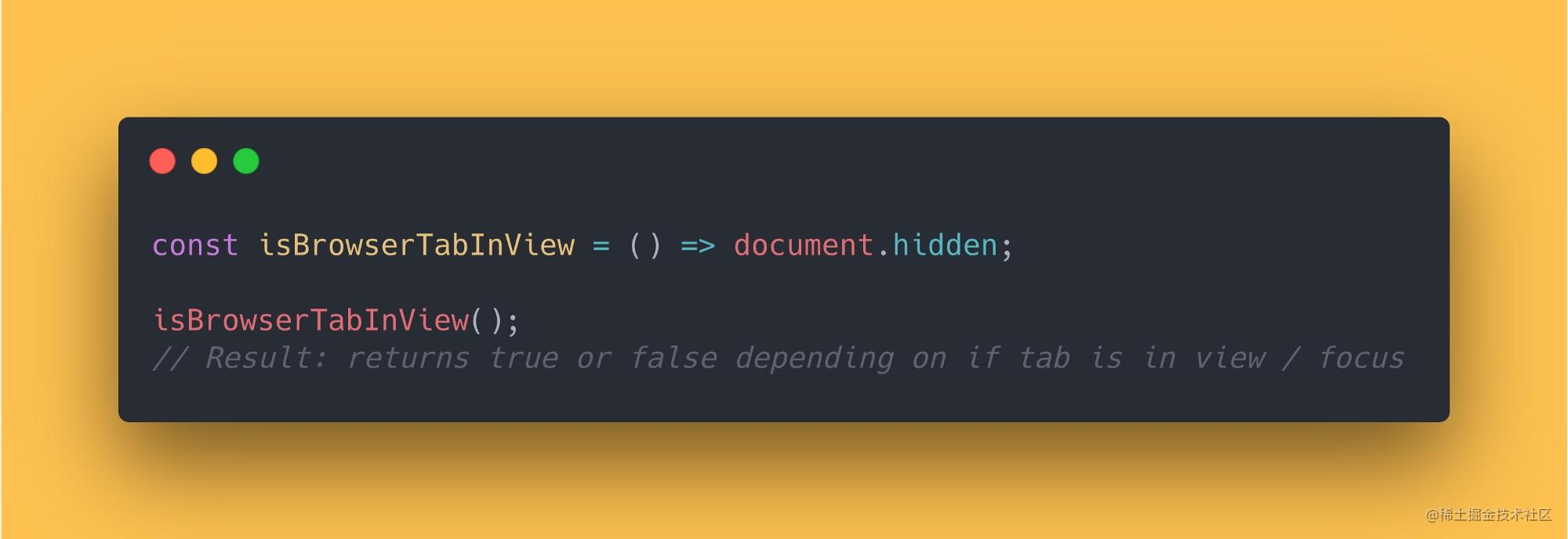``````const isBrowserTabInView = () => document.hidden;
isBrowserTabInView();
// Result: returns true or false depending on if tab is in view / focus

## 5. 检查数字是否为偶数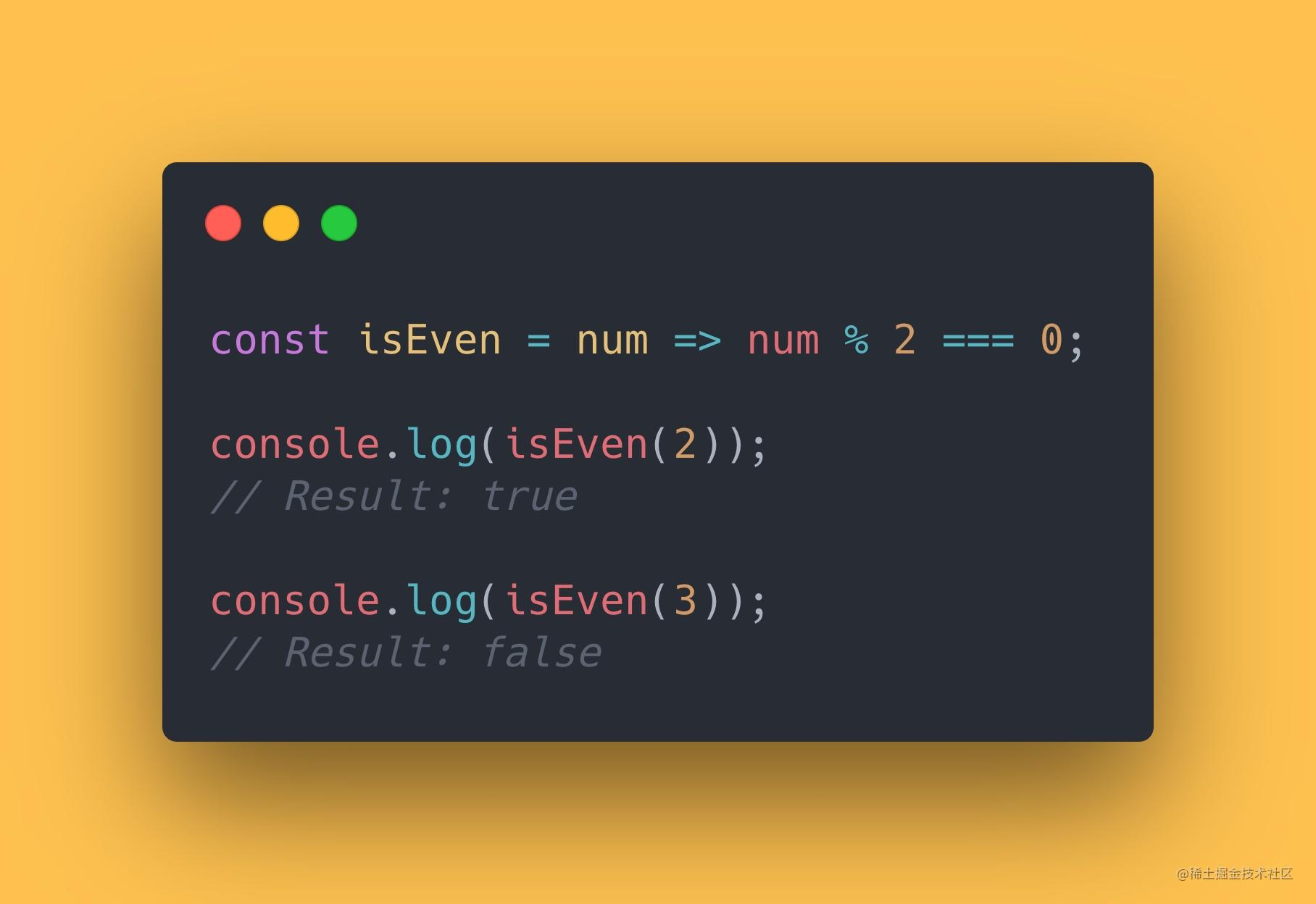``````const isEven = num => num % 2 === 0;
console.log(isEven(2));
// Result: true
console.log(isEven(3));
// Result: false

## 6. 从日期中获取时间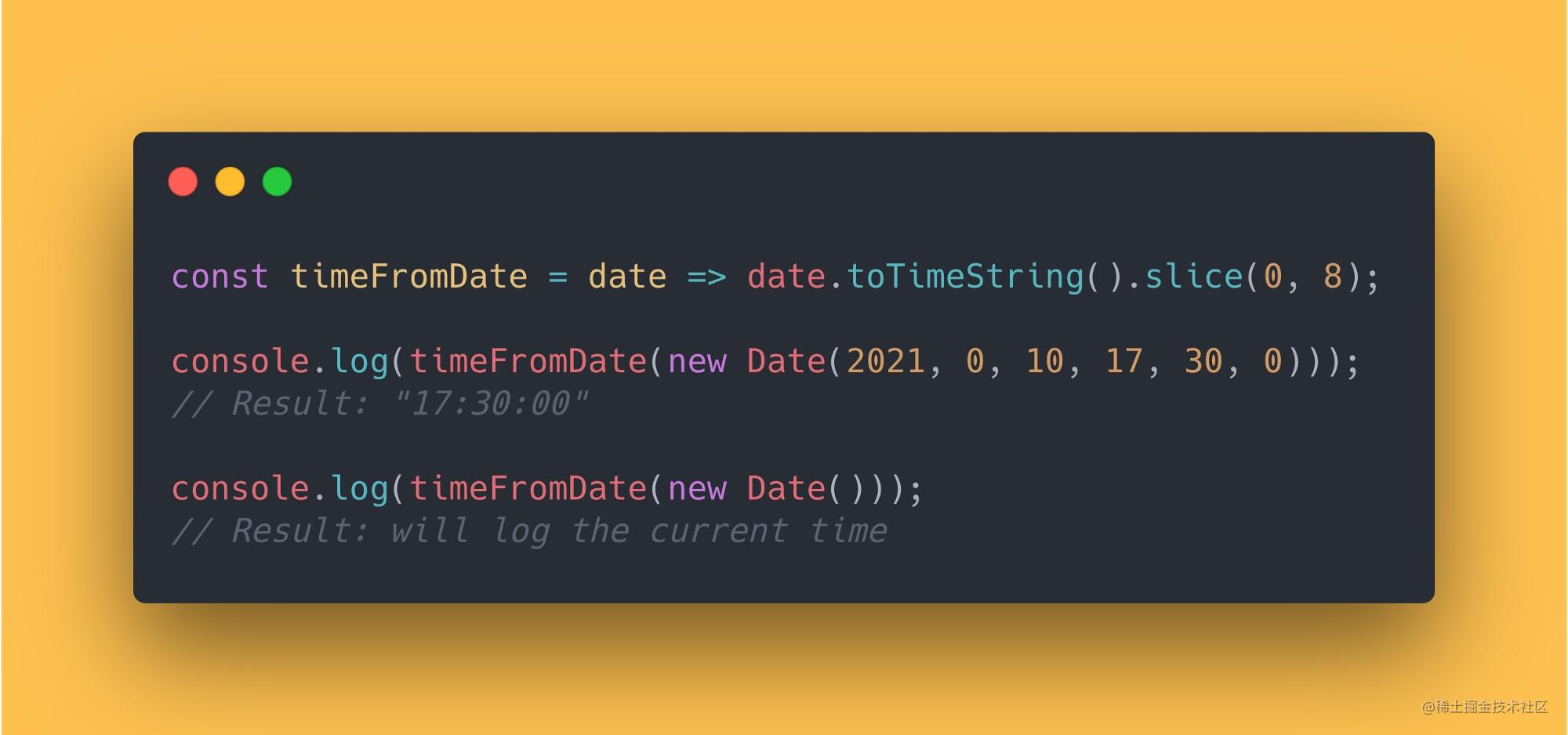``````const timeFromDate = date => date.toTimeString().slice(0, 8);
console.log(timeFromDate(new Date(2021, 0, 10, 17, 30, 0)));
// Result: "17:30:00"
console.log(timeFromDate(new Date()));
// Result: will log the current time

## 7. 保留小数点（非四舍五入）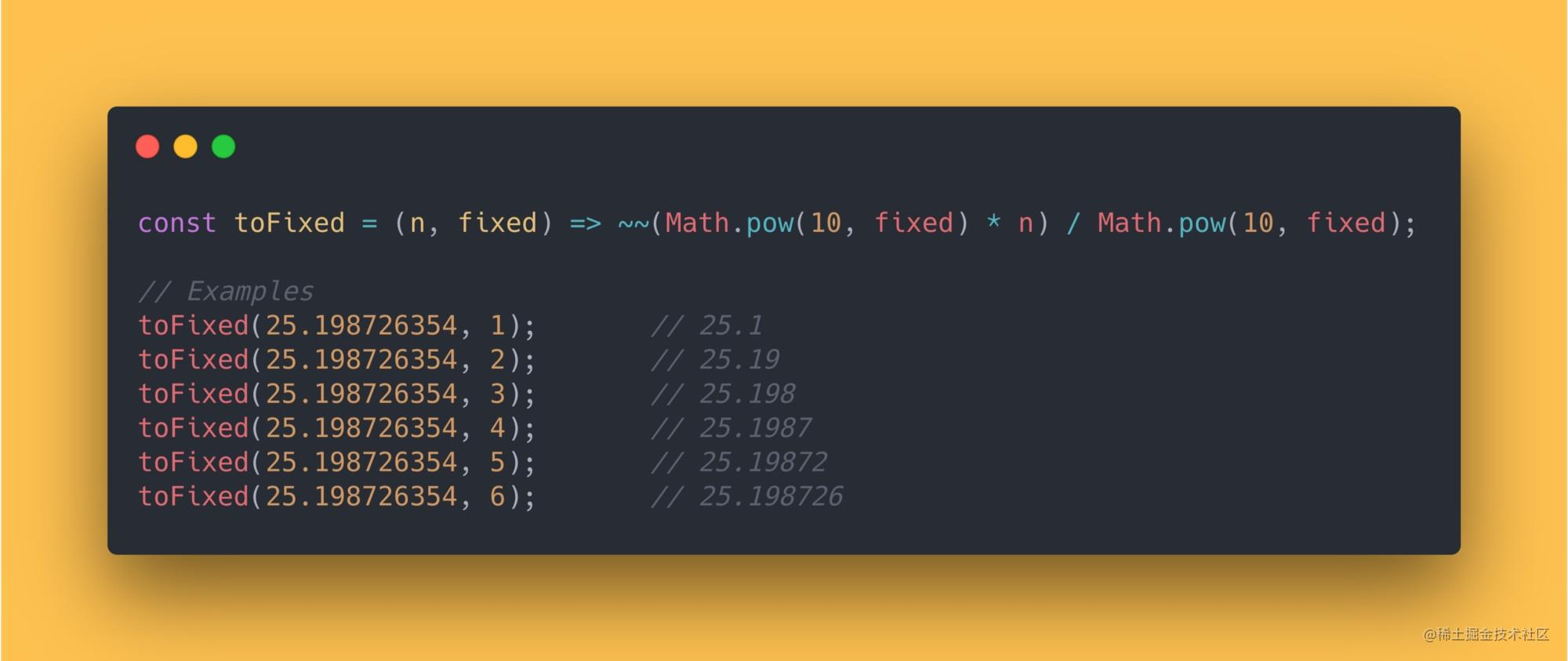``````const toFixed = (n, fixed) => ~~(Math.pow(10, fixed) * n) / Math.pow(10, fixed);
// Examples
toFixed(25.198726354, 1);       // 25.1
toFixed(25.198726354, 2);       // 25.19
toFixed(25.198726354, 3);       // 25.198
toFixed(25.198726354, 4);       // 25.1987
toFixed(25.198726354, 5);       // 25.19872
toFixed(25.198726354, 6);       // 25.198726

## 8. 检查元素当前是否为聚焦状态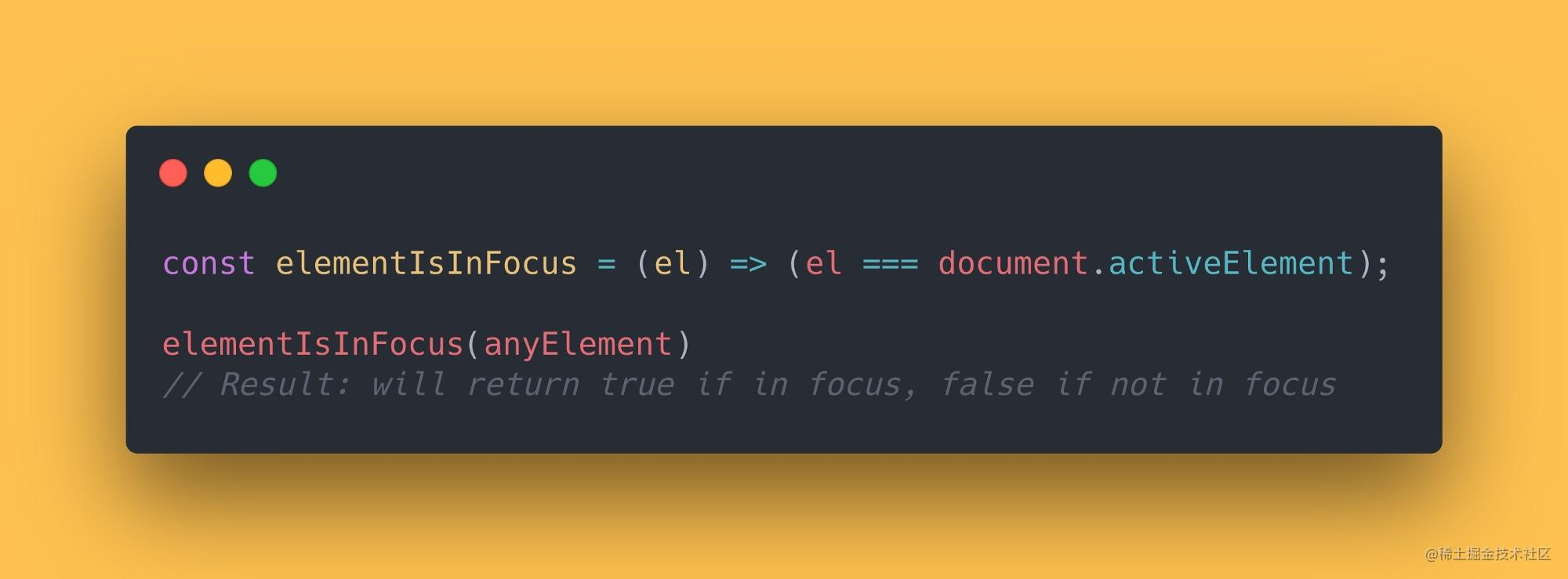``````const elementIsInFocus = (el) => (el === document.activeElement);
elementIsInFocus(anyElement)
// Result: will return true if in focus, false if not in focus

## 9. 检查浏览器是否支持触摸事件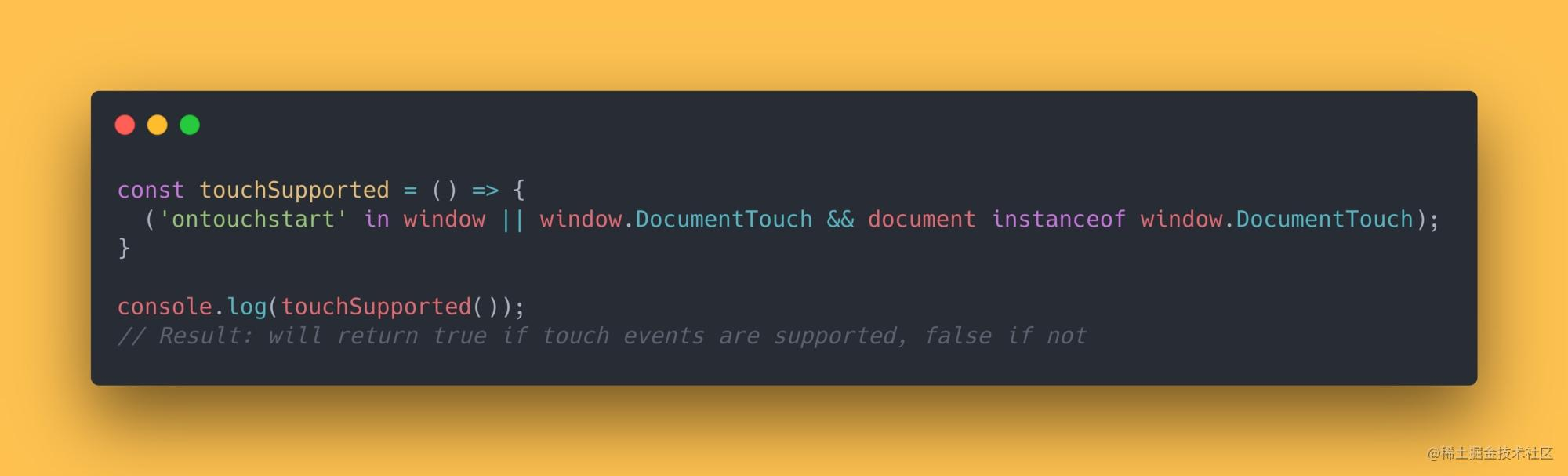``````const touchSupported = () => {
('ontouchstart' in window || window.DocumentTouch && document instanceof window.DocumentTouch);
}
console.log(touchSupported());
// Result: will return true if touch events are supported, false if not

## 10. 检查当前用户是否为苹果设备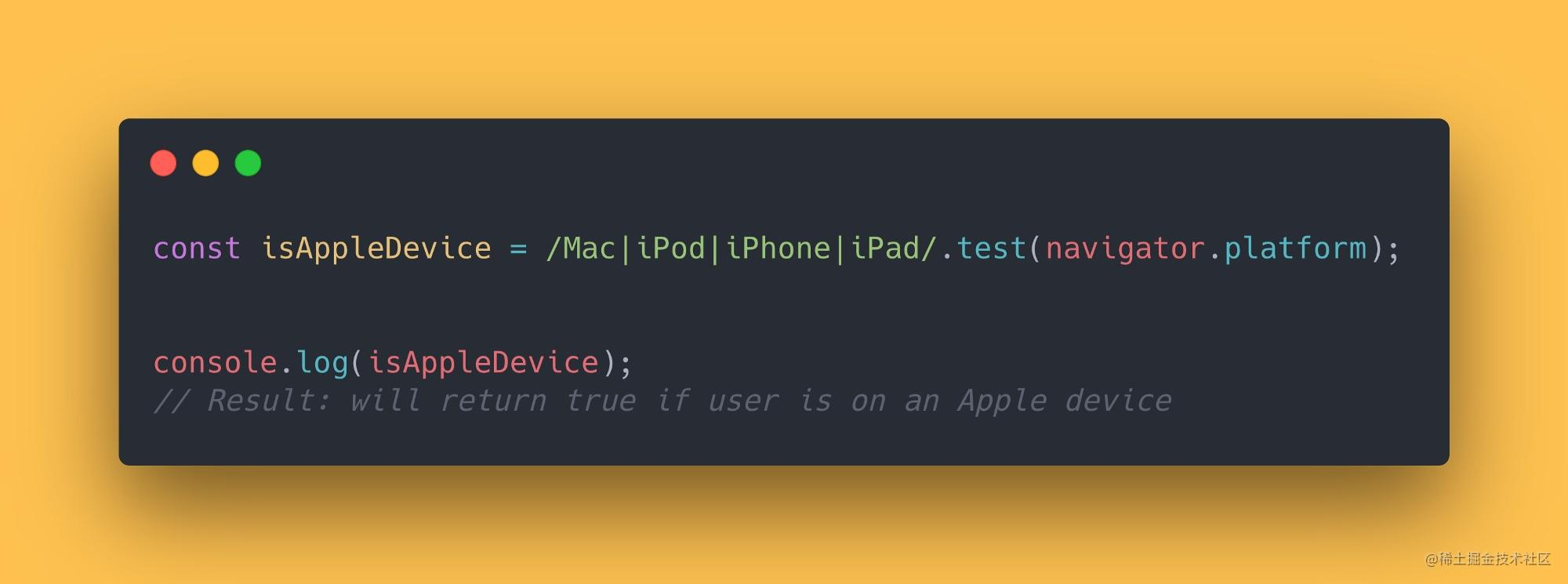``````const isAppleDevice = /Mac|iPod|iPhone|iPad/.test(navigator.platform);
console.log(isAppleDevice);
// Result: will return true if user is on an Apple device

## 11. 滚动到页面顶部

`window.scrollTo()` 方法会取一个 x 和 y 坐标来进行滚动。如果我们将这些坐标设置为零，就可以滚动到页面的顶部。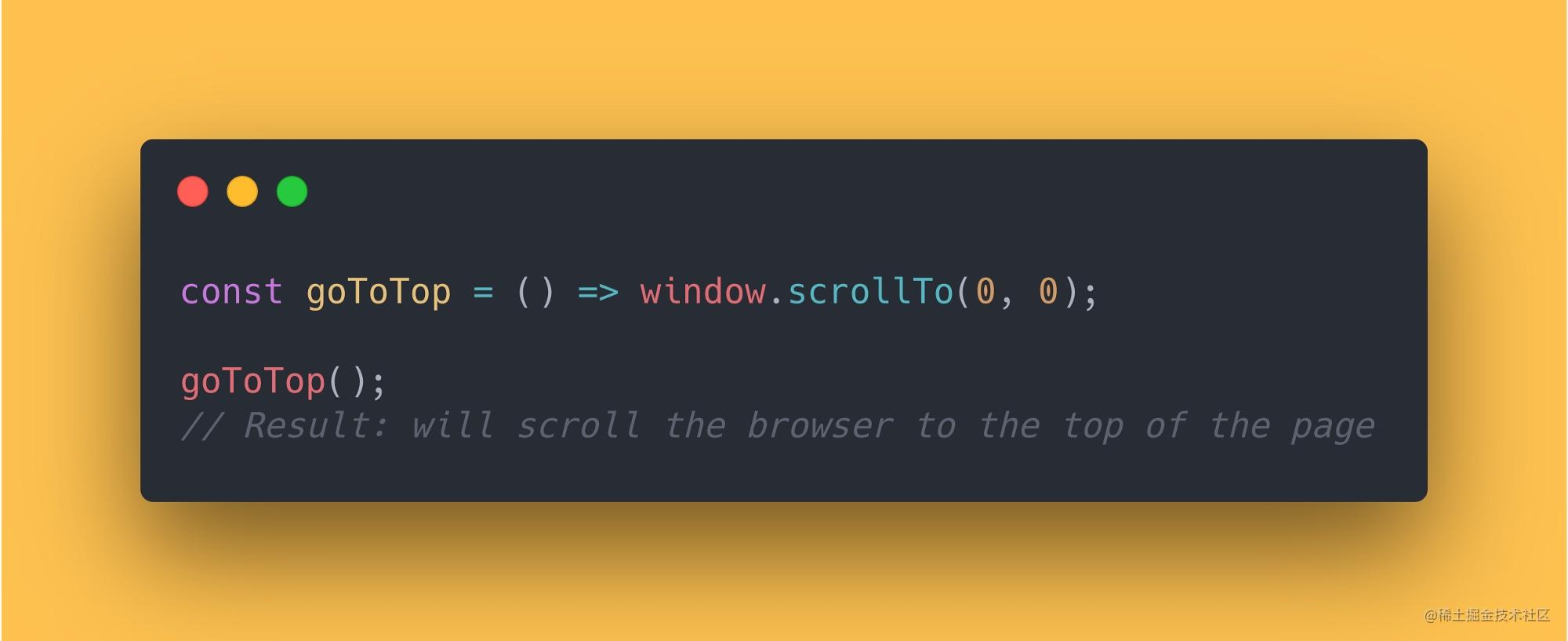``````const goToTop = () => window.scrollTo(0, 0);
goToTop();
// Result: will scroll the browser to the top of the page

## 12. 获取所有参数平均值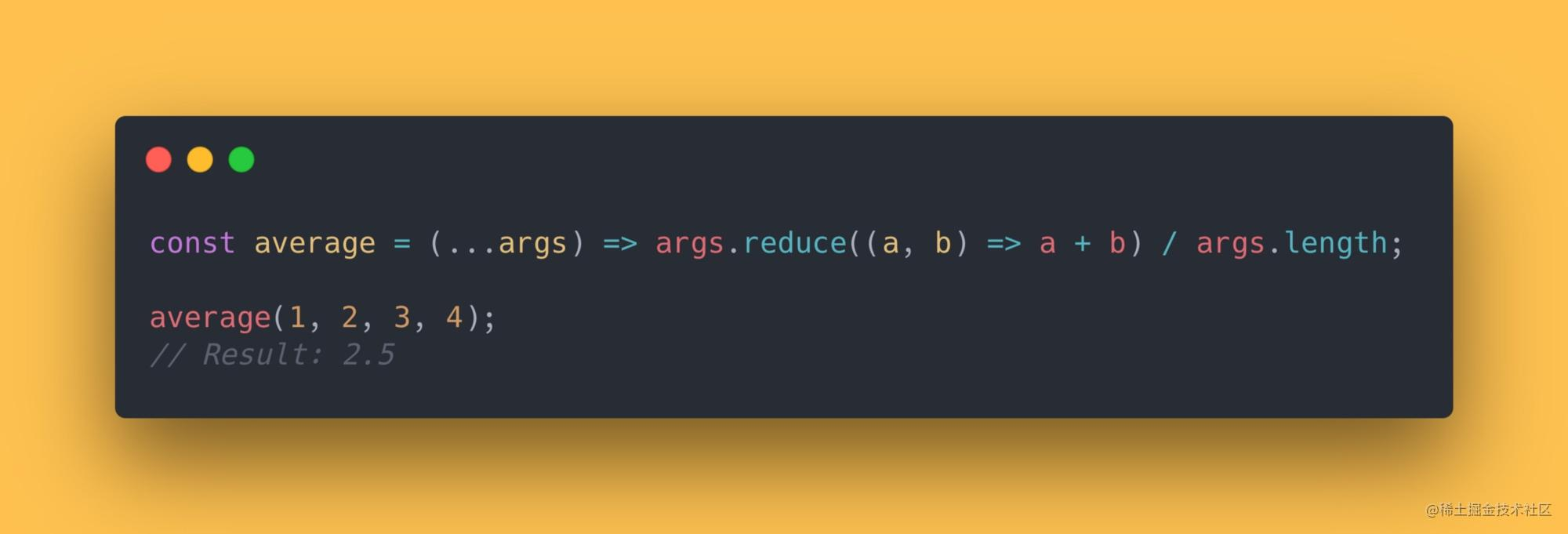``````const average = (...args) => args.reduce((a, b) => a + b) / args.length;
average(1, 2, 3, 4);
// Result: 2.5

## 13. 转换华氏度/摄氏度。（这个应该很少在国内用到吧）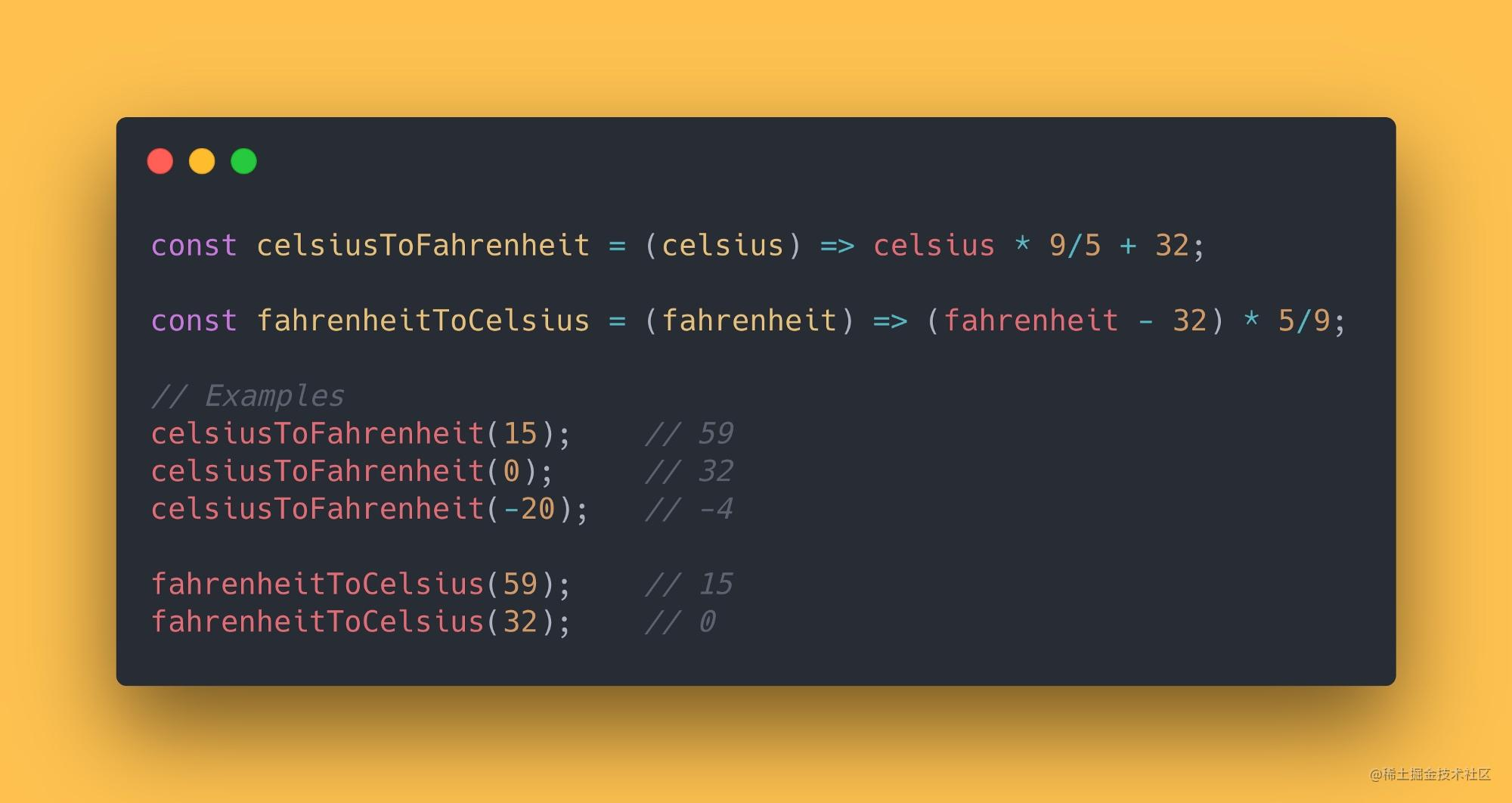``````const celsiusToFahrenheit = (celsius) => celsius * 9/5 + 32;
const fahrenheitToCelsius = (fahrenheit) => (fahrenheit - 32) * 5/9;
// Examples
celsiusToFahrenheit(15);    // 59
celsiusToFahrenheit(0);     // 32
celsiusToFahrenheit(-20);   // -4
fahrenheitToCelsius(59);    // 15
fahrenheitToCelsius(32);    // 0

• yck
2年前
• Neal_yang
3年前
• Gaby
1年前
• vortesnail
1年前
• 徐小夕
2年前
•• 伊人a
1年前• CUGGZ
2年前• LBJ
1年前